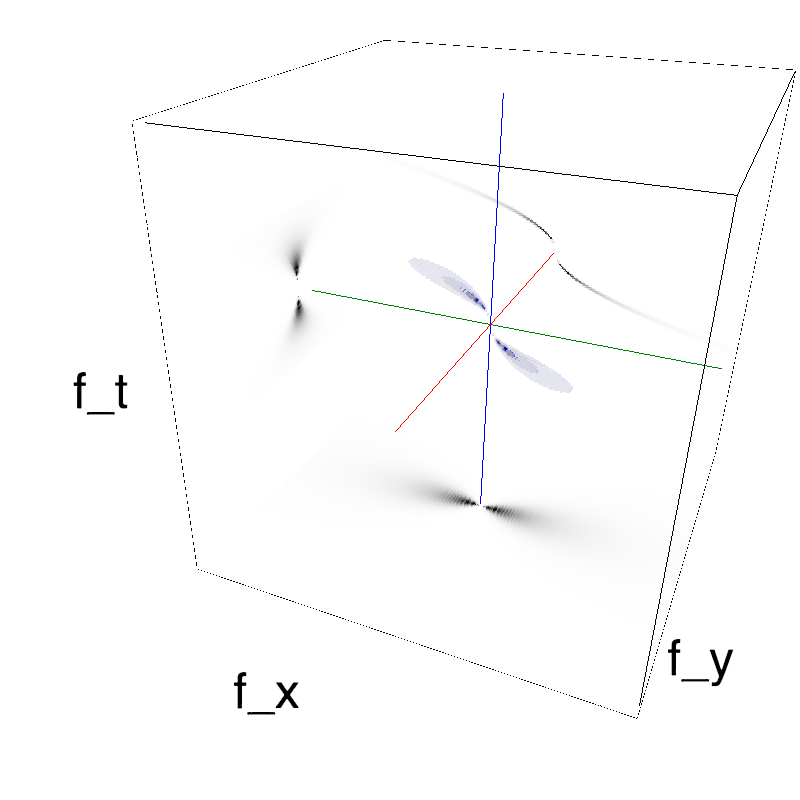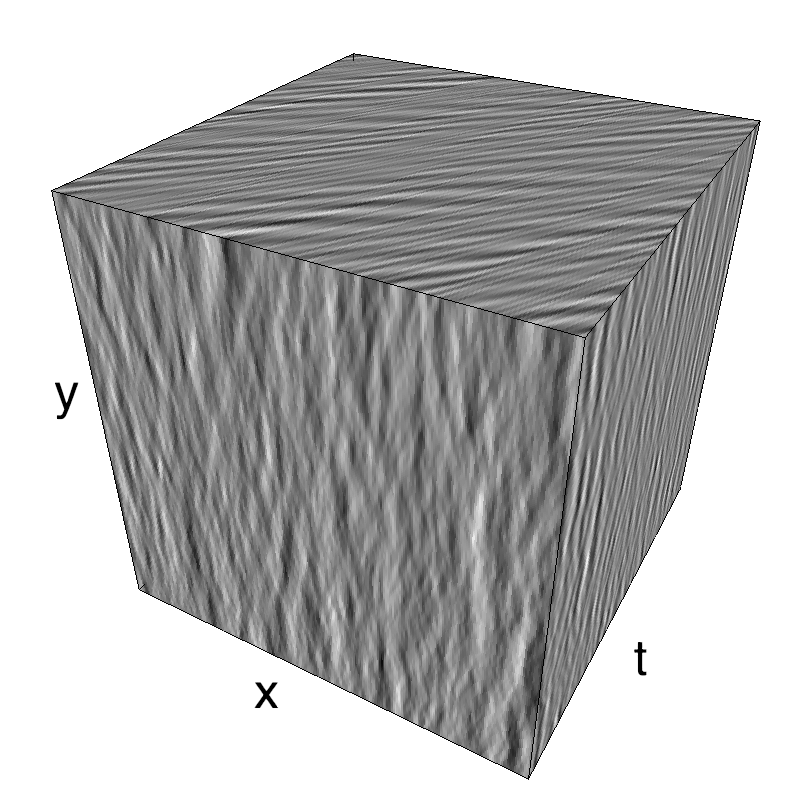# A bit more fun with gravity waves

## More fun with gravity waves¶

Motion Clouds were defined in the origin to provide a simple parameterization for textures. Thus we used a simple unimodal, normal distribution (on the log-radial frequency space to be more precise). But the larger set of Random Phase Textures may provide some interesting examples, some of them can even be fun! This is the case of this simulation of the waves you may observe on the surface on the ocean.

Main features of gravitational waves are:

1. longer waves travel faster (tsunami are fast and global, ripples are slow and local) - speed is linearly proportional to wavelength
2. phase speed (following a wave's crest) is twice as fast as group speed (following a group of waves).

In :
```import os
import numpy as np
import MotionClouds as mc
fx, fy, ft = mc.get_grids(mc.N_X, mc.N_Y, mc.N_frame)
mc.figpath = '../files/2016-04-24_a-wave-going-backwards'
if not(os.path.isdir(mc.figpath)): os.mkdir(mc.figpath)

def envelope_gravity(fx, fy, ft, B_wave, V_X, V_Y, g=.1):
"""
Gravitational envelope:
selects the manifold corresponding to the speed (V_X, V_Y) with some thickness B_V

"""
k = fx*V_X+fy*V_Y
env = np.exp(-.5*(((ft/.5)**2-g*np.sqrt(((k/.5)**2)))**2/(B_wave*mc.frequency_radius(fx, fy, ft, clean_division=True))**2))
env *= (ft*k) < 0
return env

def envelope_gabor_wave(fx, fy, ft, B_wave, V_X, V_Y,
B_V=mc.B_V, B_v=1., sf_0=mc.sf_0, B_sf=mc.B_sf, loggabor=mc.loggabor,
theta=mc.theta, B_theta=mc.B_theta, alpha=mc.alpha):
"""
Returns the Motion Cloud kernel

"""
envelope = mc.envelope_gabor(fx, fy, ft, V_X, V_Y,
B_V=B_V, sf_0=sf_0, B_sf=B_sf, loggabor=loggabor,
theta=theta, B_theta=B_theta, alpha=alpha)
envelope *= envelope_gravity(fx, fy, ft, B_wave=B_wave, V_X=V_X, V_Y=V_Y)
return envelope
```
In :
```name = 'wave2'
theta, B_theta, B_wave = 0., np.pi/12., .02
alpha, sf_0, B_sf, B_V = 1., .2, .4, 2.
recompute = True
env = envelope_gabor_wave(fx, fy, ft, V_X=1.5, V_Y=0., B_wave=B_wave, B_V=B_V,
theta=theta, B_theta=B_theta, sf_0=sf_0, B_sf=B_sf, alpha=alpha)
mc.figures(env, name, recompute=recompute, figpath=mc.figpath)
mc.in_show_video(name, figpath=mc.figpath)
```
```/usr/local/lib/python3.6/site-packages/vispy/visuals/isocurve.py:22: UserWarning: VisPy is not yet compatible with matplotlib 2.2+
warnings.warn("VisPy is not yet compatible with matplotlib 2.2+")
```In :
```name = 'wave2_back'
im = mc.rectif(mc.random_cloud(env))
```
In :
```im_back = im.copy()
for i in range(mc.N_frame):
im_back[:, :, i] = np.roll(im[:, :, i], -i, axis=0)
mc.anim_save(im_back, os.path.join(mc.figpath, name))
mc.in_show_video(name, figpath=mc.figpath)
```

### Post-prod¶

In :
```!curl https://upload.wikimedia.org/wikipedia/commons/thumb/4/4f/Paperboat.svg/1000px-Paperboat.svg.png -o /tmp/Paperboat.png
```
```  % Total    % Received % Xferd  Average Speed   Time    Time     Time  Current
100 38858  100 38858    0     0   221k      0 --:--:-- --:--:-- --:--:--  220k
```
In :
```# Import everything needed to edit video clips
import moviepy.editor as mvp
w,h = moviesize = (mc.N_X, mc.N_Y)
fps = 25
zoom = 1.5
duration = mc.N_frame/fps
clip = mvp.VideoFileClip(os.path.join(mc.figpath, name+mc.vext))
clip.ipython_display(fps=fps, duration=duration, autoplay=1, loop=1)
```
```pygame 1.9.4
Hello from the pygame community. https://www.pygame.org/contribute.html
```
```100%|█████████▉| 256/257 [00:00<00:00, 943.70it/s]
```
Out: